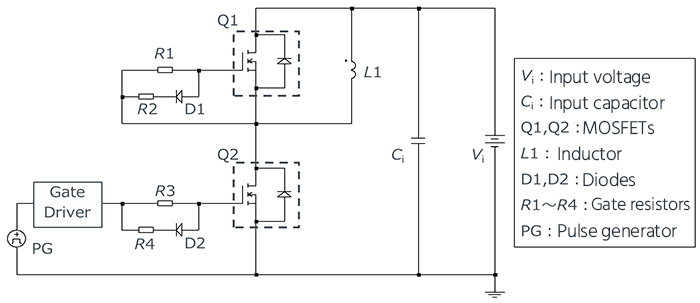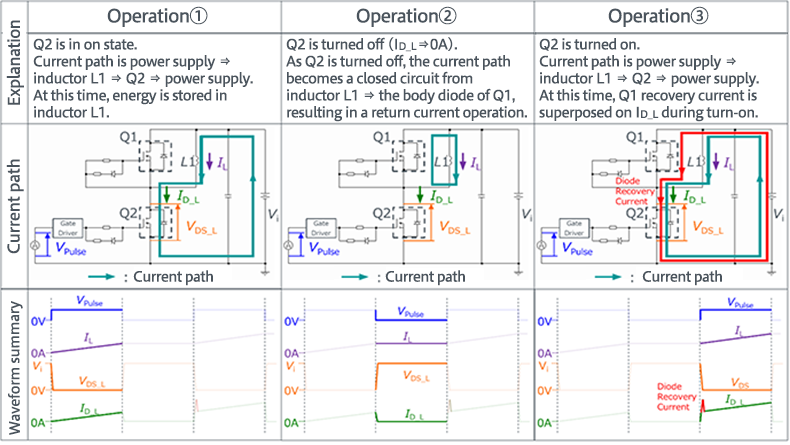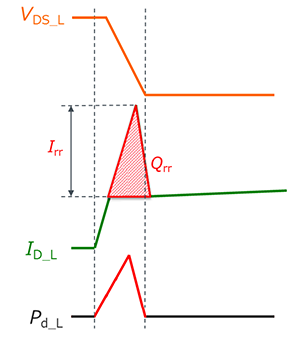Technical Information Site of Power Supply Design

2021.06.23 Si Power Device

Evaluating MOSFET Recovery Characteristics Using Double-Pulse Tests

A new section on "Evaluation" of Si power devices has been created. In "Evaluating MOSFET Recovery Characteristics Using Double-Pulse Tests", the recovery characteristics of MOSFET body diodes are evaluated by double-pulse tests, and losses are considered.

The Relationship Between MOSFET Body Diode Recovery Characteristics and Bridge Circuit Losses

In bridge circuits that use two or more MOSFETs such as inverter circuits and totem pole-type power factor correction (PFC) circuits, turn-on losses may increase due to currents passing through the upper and lower arms. This phenomenon is greatly affected by the recovery characteristics of the body diode (parasitic diode) of the MOSFET in the opposite arm to the MOSFET that is switching. Hence in a bridge circuit, MOSFETs with excellent body diode recovery characteristics are superior.

Double-pulse testing is a method widely used to evaluate the characteristics of power switching elements such as MOSFETs and IGBTs. In these tests, apart from the switching characteristics of the tested element, it is also possible to evaluate the recovery characteristics of the body diode and fast recovery diodes (FRDs) which are often used together with IGBTs. Hence these tests are extremely useful for evaluations that assume a circuit in which losses occur due to recovery characteristics during driven-side element turn-on. The basic circuit diagram for double-pulse tests is shown below.The table shown below indicates the basic operation of double-pulse tests when, in the above circuit, Q1 is a free-wheeling MOSFET and Q2 is a driven-side MOSFET. The basic operation can be mainly categorized as three modes ①, ②, and ③. The operation, current paths, and waveforms in each of these modes are shown for a pulse generator voltage VPulse, inductor current IL, Q2 drain-source voltage VDS_L, and Q2 drain current ID_L.In operation ③, a short-circuit current (the red part of ID_L) is observed when Q2 is turned on. This occurs due to the recovery characteristic of the Q1 body diode.

When a body diode transitions from on to off, the charge accumulated while the diode was on must be discharged. If the amount of charge discharged from the body diode at this time is Qrr, the peak value of the current resulting from the discharge is Irr, and the power loss in Q2 is Pd_L, then the operation of turning on Q2 can be represented as in the figure on the right. The area of the triangular shape described by ID_L is Qrr, and the height of the triangle is Irr.In general, when the recovery characteristics of the body diode of the free-wheeling element Q1 are poor and Qrr is large, the turn-on loss in the driven-side element Q2 increases. Consequently, in the case of an application in which a free-wheeling current flows such as an inverter circuit or in a totem pole-type PFC circuit, it is necessary to consider the substantial influence that the body diode recovery characteristic has on losses.

#### Key Points:

・In a bridge circuit with two or more MOSFETs, turn-on losses are higher when the recovery characteristics of the body diodes in the MOSFETs are poor.

・Double-pulse testing is a widely used method for evaluating the characteristics of power switching elements such as MOSFETs and IGBTs.

・In addition to the switching characteristics of a tested element, the recovery characteristics of the body diode and of external fast recovery diodes and the like can also be evaluated.

・Double-pulse tests are extremely useful for circuits in which losses arise due to recovery characteristics while a driven-side element is turned on.